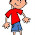## Sunday, October 2, 2011

### Python, statistics: Ranking a single array of raw scores

There are some nonparametric statistical routines which expects rank values. If we are given raw scores
like X= [1,3,3,5,1,7], the rank values would be [1,2,2,3,1,4] if tied ranks get the same rank value.

But the ranks can be transformed to [0.5, 2.5, 2.5,3, .5, 4] if ties are replaced by the mean of tied ranks.

Here is Python code to compute ranks based on raw scores according to four strategies published in the reference.

"""
File      scores2ranks.py
UPDEPP at Clarkfield, Pampanga
Desc      Conversion of raw scores to ranks using various strategies.
Version   0.0.1 October 1, 2011
Reference http://en.wikipedia.org/wiki/Ranking
"""

def scores2ranks(X, ztol = 1.0e-1, breakties = 1):
"""
Converts raw scores to ranks, returning an array of ranks.
Args
X - scores to convert to ranks
ztol - equality comparison tolerance
breakties- strategy:
0 - None.                               1234            ordinal ranking
1 - replace  ties by mean of tied ranks.1 2.5, 2.5, 4 fractional ranking.
2 - (competition rank)                  1224            standard competition ranking.
3 - replace ties by highest tied rank.  1334            modified competition ranking.
4 - replace rank after ties in sequence 1223            dense ranking.
References: For conversion of matrix scores to ranks using fractional ranking:
http://my-other-life-as-programmer.blogspot.com/2011/02/python-converting-raw-scores-to-ranks.html
"""
Z = [(x, i) for i, x in enumerate(X)]
Z.sort()
n = len(Z)
Rx =  * n
for j, (x,i) in enumerate(Z):
Rx[i] = j+1
if breakties == 0:
return Rx
s = 1           # sum of ties.
start = end = 0 # starting and ending marks.
for i in range(1, n):
if abs(Z[i] -Z[i-1]) < ztol and i != n-1:
pos = Z[i]
s+= Rx[pos]
end = i
else: #end of similar x values.
if breakties == 1:
tiedRank = float(s)/(end-start+1)
for j in range(start, end+1):
Rx[Z[j]] = tiedRank
if breakties == 2 or  breakties == 4:
tiedRank = Rx[Z[start]]
for j in range(start, end+1):
Rx[Z[j]] = tiedRank
if breakties == 3:
tiedRank = Rx[Z[end]]
for j in range(start, end+1):
Rx[Z[j]] = tiedRank
start = end = i
s = Rx[Z[i]]

if breakties == 4:
#ensure that  the ranks are in sequence!
for i, x in enumerate(sorted(list(set(Rx[:])))):
for j, y in enumerate(Rx):
if y == x:
Rx[j] = i+1
return Rx

if __name__ == "__main__":
X= [1,3,3,5,  1,  7]
print "X = ", X
print scores2ranks(X,  breakties = 4)

When the above code is run, it outputs
\$ python scores2ranks.py
X =  [1, 3, 3, 5, 1, 7]
strategy 0 : [1, 3, 4, 5, 2, 6]
strategy 1 : [1.5, 3.5, 3.5, 5.0, 1.5, 6]
strategy 2 : [1, 3, 3, 5, 1, 6]
strategy 3 : [2, 4, 4, 5, 2, 6]
strategy 4 : [1, 2, 2, 3, 1, 4]


I will be grateful if readers will discover any mistake.

1.2.